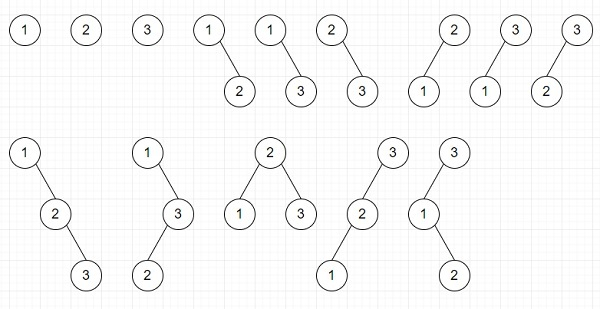# Program to find number of possible BSTs can be generated using n distinct nodes in Python

Suppose we have a number n. If we have numbers like [1,2,...,n] we have to count number ofpossible BSTs can be formed using these n values. If the answer is too large, then mod the result by 10^9+7.

So, if the input is like n = 3, then the output will be 14,To solve this, we will follow these steps

• a := a list with values [0, 1]
• m := 10^9+7
• max_n := 1000
• for k in range 2 to max_n + 1, do
• insert (1 + sum of all elements of the list (a[i] * a[k - i] for all i in range(1, k))) mod m at the end of a
• return (a[n + 1] - 1) mod m

## Example

Let us see the following implementation to get better understanding −

def solve(n):
a = [0, 1]
m = 10**9+7
max_n = 1000

for k in range(2, max_n + 2):
a.append((1 + sum(a[i] * a[k - i] for i in range(1, k))) % m)
return ((a[n + 1] - 1) % m)

n = 3
print(solve(n))

## Input

3


## Output

14